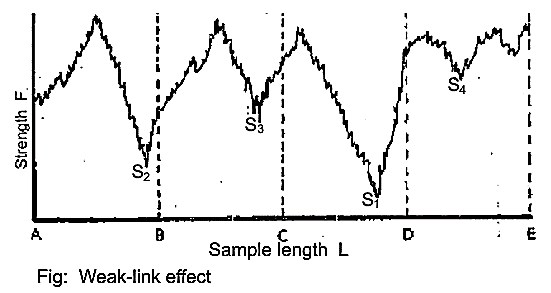1.33K
0#### Md Sohanur Rahman Sobuj

Co-founder , Admin & Author at Textile Study Center
Student of Bangladesh University of Textiles (BUTEX) Department: Apparel Engineering#### Latest posts by Md Sohanur Rahman Sobuj (see all)

In general, the effect of sample length on breaking strength has been shown that the mean measured the strength of a specimen decreases as the sample length is increased. This fact is well known as the “weak-link” effect.

Suppose that the yarn strength at every point along the length is like Figure. If the sample lengths are AB, BC, CD, and DE, the measured strengths should be S2, S3, S1, and S4 respectively, which are the weakest places in each    sample length, and the mean strength is the average(S’) of S2, S3, S1, and S4. But if the sample is tested at twice the length, AC and CE, each will break at its own weakest place, S2 and S1, and the mean strength is the average(S”) of S2 and S1. Further if the sample is tested at length AE, the strength must be S1.

In this way, the mean strength decreases with the increase of sample length.

The weak-link effect described the following results:

The mean measured strength of a specimen decreases as the test-length is increased.

The decrease in mean measured strength will be more rapid the more irregular the fiber is. (S”-S’=difference­, irregularity­)

The order of ranking of specimens may alter if the test-length is altered.

(1332)#### Md Sohanur Rahman Sobuj

Co-founder , Admin & Author at Textile Study Center
Student of Bangladesh University of Textiles (BUTEX) Department: Apparel Engineering#### Latest posts by Md Sohanur Rahman Sobuj (see all)Close
Share via